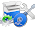# Data Structure and Algorithm Analysis Set 7

## Data Structure and Algorithm Analysis

### Questions 61 to 70

61.
List out the areas in which data structures are applied extensively.
 (a) Compiler Design (b) Operating System (c) Database Management System (d) Both (a) and (b) above (e) (a), (b) and (c) above.
62.
What is the major data structure used in the Network data model?
 (a) Stack (b) Array (c) Tree (d) Graph (e) Queue.
63.
The data structure used in the Hierarchical data model is
 (a) Array (b) Tree (c) Graph (d) Stack (e) Queue.
64.
Minimum number of queue(s) needed to implement the priority queue is(are)
 (a) Two (b) One (c) Three (d) Four (e) Depends upon the application.
65.
What data structure is used to perform recursion?
 (a) Queue (b) Linked List (c) Stack (d) Double Linked List (e) Circular Queue.
66.
For the expression ((A + B) * C – (D – E) ^ (F + G)), the equivalent Postfix notation is
 (a) AB + C * DE - - ^ FG + (b) AB + CDE - - * FG + ^ (c) AB + C * DE - FG + - ^ (d) AB + C * DE - - FG + ^ (e) AB + C - DE - * FG + ^.
67.
The Prefix notation for the above infix expression is
 (a) - * +ABC - DE ^ + FG (b) ^ - * +ABC - DE + FG (c) ^ - +AB * C - DE + FG (d) ^ - + * ABC - DE + FG (e) - ^ * + ABC - DE + FG.
68.
Sorting is not possible by using which of the following methods?
 (a) Insertion (b) Selection (c) Deletion (d) Exchange (e) Partitioning.
69.
The number of null branches for a binary tree with 20 nodes is
 (a) 21 (b) 20 (c) 22 (d) 19 (e) 18
70.
What is the type of the algorithm used in solving the 8 Queens problem?
 (a) Greedy (b) Dynamic (c) Branch and Bound (d) Divide and Conquer (e) Backtracking.

#### Answers

 61 Answer : (e) Reason : As the data structure can be used in all the application areas right from the compiler design to operating systems and even in database management system design. 62 Answer : (d) Reason : As the graph is the suitable data structure to represent the connectivity between the nodes as edges. 63 Answer : (b) Reason : As tree data structure is used to represent the Hierarchical relationship between the nodes as parent and children. 64 Answer : (a) Reason : Two : One queue is used for actual storing of data and another for storing priorities. 65 Answer : (c) Reason : Because of its LIFO (Last In First Out) property it remembers its ‘caller’ so knows whom to return when the function has to return. Recursion makes use of system stack for storing the return addresses of the function calls. 66 Answer : (d) Reason : As per the precedence and associativity of the operators, brackets are evaluated first and then multiplication operators followed by addition, subtraction operators. 67 Answer : (b) Reason : As per the precedence and associativity of the operators, brackets are evaluated first and then multiplication operators followed by addition, subtraction operators. 68 Answer : (c) Reason : Using insertion we can perform insertion sort, using selection we can perform selection sort, using exchange we can perform the bubble sort (and other similar sorting methods) and using the portioning we can perform merge sort(and other similar sorting methods) But no sorting method can be done just using deletion. 69 Answer : (a) Reason : A binary tree with n nodes has exactly n+1 null nodes. 70 Answer : (e) Reason : As the other types of algorithms are not suitable to find the solution for the given problem.

<< PrevNext >>

#### 5 comments :

1.very good and very useful

2.3.Programming is very interesting and creative thing if you do it with love. Your blog code helps a lot to beginners to learn programming from basic to advance level. I really love this blog because I learn a lot from here and this process is still continuing.
Love from Pro Programmer

4.Very Useful Post!Thanks!
Data Structure Programming

5.This is an informative post and it is very useful and knowledgeable. therefore, I would like to thank you for the efforts you have made in writing this article.
apple ios training institutes in Hyderabad
ios app development in hyderabad